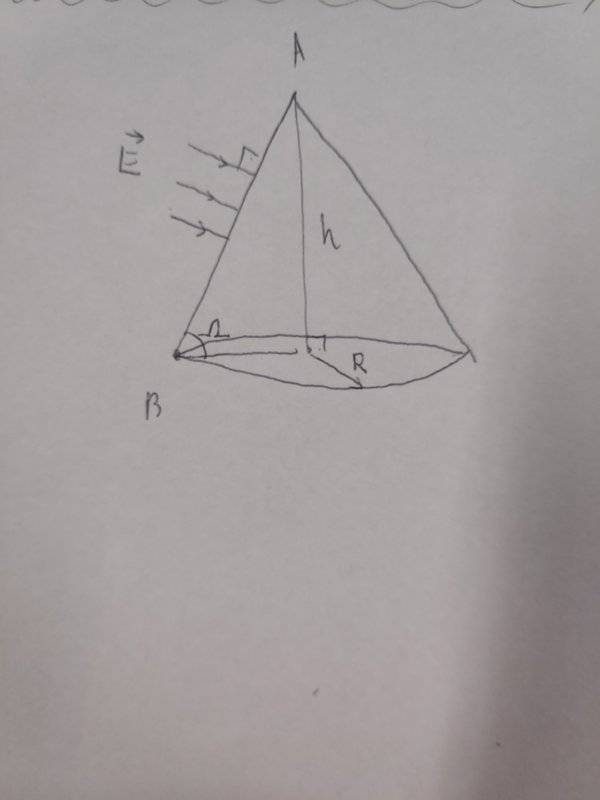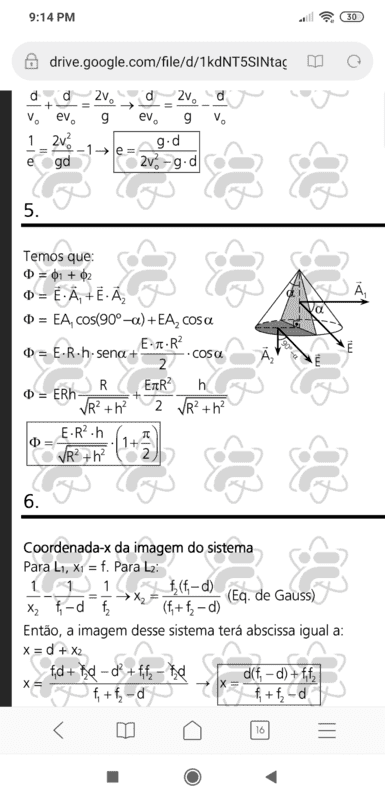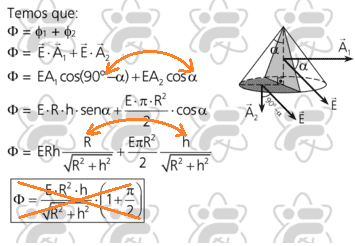# Flux of Electric Field through a cone

Homework Statement:
A conic surface is placed Inside a region filled with uniform Electric Field E. The field lines are perpendicular to the geratrix AB. The cone has height h and radius R of it's base. Find the electric flux through the lateral surface of the cone.
Relevant Equations:
Ø=E.S.cosx Øtot=qint/€ (gaus law)
Since there is no charge inside the cone, the total flux through its surface is zero, hence Ø(lateral surface)+∅(base surface)=0. But ∅(base surface)=E.πR².cosΩ, because electric Field is homogenous. But by the figure, Ω is just arctg(h/R).
So Ø(lateral surface)=-E.π.R².R/√(R²+h²).
This is not the answer according to the solution I saw. My point is, does anyone could show me where is my mistake?TSny
Homework Helper
Gold Member
Your work looks correct to me. Do you remember the form of the answer from the solution?

Here is the solution I saw. The sentence : " temos que" is equal to " we have that"TSny
Homework Helper
Gold Member
Looks like this is a different problem where they want the flux through the two shaded regions (the triangle and the semicircle). But I think there is a mistake in the solution where they inadvertently switched the two angles in the third line:Anyway, I think your work is correct for the problem you stated.

•Moara